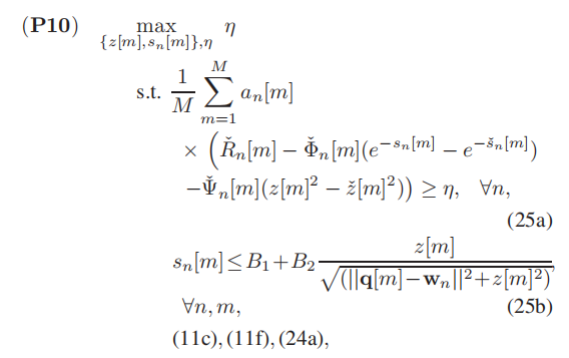# How to make x/norm([x a]) feasible?

here’s my code.
elevation = cvx(zeros(n,m));
for i = 1:n
for j = 1:m
elevation(i,j) = UAV_vertical_trajectory(1,j)/norm( [hor_distance0( i,j) UAV_vertical_trajectory( 1,j )] );
end
end

Where UAV_vertical_trajectory is a 1m matrix variable ,and hor_distance0 is a known nm matrix.
When I run the code,the result I get is :Disciplined convex programming error:
Cannot perform the operation: {real affine} ./ {convex}

When a ~= 0, the 2nd derivative of `x/sqrt(x^2+a^2)` is positive for negative x, and negative for positive x. Hence it is neither convex nor concave. Therefore, it can’t be used in CVX.

Of course, we don;t know what you are trying to do. For instance if you are trying to model a constraint `x/norm([x a]) >= 1`, you can multiply that out to `x >= norm([x a])`, which is a convex constraint, and is accepted by CVX.

I commend you to carefully read

dear Mark,thanks for you help,after getting reply i have read (why isn’t CVX accepting my model?) carefully.I am sure my model is convex. and i have done many attempts.but i still haven’t solved my problem.
in fact,UAV_vertical_trajectory(1,j) is a variable greater than 200.When I wrote 25b, I had a big problem.in 25b,sn[m] is a variable nm matrix,and z[m] is a 1m variable matrix.B1 B2 qm wn are all known.and B1<0,B2>0.
i have tried to move B1 to left side and square both sides,but i faild.
and i tried to write it with
for i = 1:n
for j = 1:m
B2*UAV_vertical_trajectory(1,j)*inv_pos(index(i,j)-B1)>=norm([UAV_vertical_trajectory(1,j) distance0(i,j)]);
end
end
but i faild again. I sincerely hope for your help

You wrote:

I am sure my model is convex

Constraint (25b) does not look convex to me. Please show us your proof that it is.

I don’t see any constraint forcing `z` to be >= 200.(or, in any event, positive). And even if there were, it is usually not possible to reformulate within DCP rules for functions which are not convex (or concave) over their entire natural domain…